×

Search anything:

# Cuckoo filter [Explained]

#### Data Structures probabilistic algorithm CultureGet this book -> Problems on Array: For Interviews and Competitive Programming

A cuckoo filter is a probabilistic data structure that is used to test whether an element is a member of a set or not. It is similar to Bloom filter. It can show false positives but not false negatives. It can also delete existing items.

It is a hash table that uses cuckoo hashing to resolve collisions. It decreases the space used by only maintaining a fingerprint of the value to be stored in the set. It uses a small f-bit fingerprint to store the data. The value of f is decided by the ideal false positive rate.

The false-positive rate is the probability that a false alarm will be raised. Even though the true value is negative it will give a positive result. It is calculated as FP/(FP+TN), where FP is the number of false positives and TN is the number of true negatives.

• Buckets in cuckoo filter
• Cuckoo hashing in cuckoo filter
• Insertion operation in cuckoo filter
• Lookup function in cuckoo filter
• Delete operation in cuckoo filter
• Implementing cuckoo filter
• Time & Space complexity of Cuckoo Filter
• Advantages of Cuckoo filter over Bloom filter
• Limitations of Cuckoo Filter

# Buckets in cuckoo filter

Cuckoo filter stores the fingerprint of the value to be stored in an array of buckets. The number of fingerprints that a bucket can hold is referred to as b. They have a load that describes the percentage of the filter they are currently using and plays a key role in deciding when cuckoo filter size should be resized. It uses the semi-sort technique. The semi-sort technique can compress the buckets by sorting the fingerprints present in them.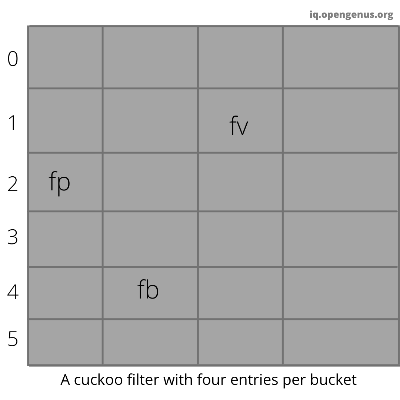# Cuckoo hashing in cuckoo filter

The cuckoo filter uses cuckoo hashing to resolve collisions. Cuckoo hashing is a method used to avoid collisions in a hash table. It got its name because of its similarities with cuckoo. As a chick of cuckoo pushes the other eggs out of the nest to make a place of their own. Similarly in cuckoo hashing, to insert a new key into the hash table we push the older key to a new place. Though cuckoo filter is based on cuckoo hashing, they use a derivative called partial-key cuckoo hashing.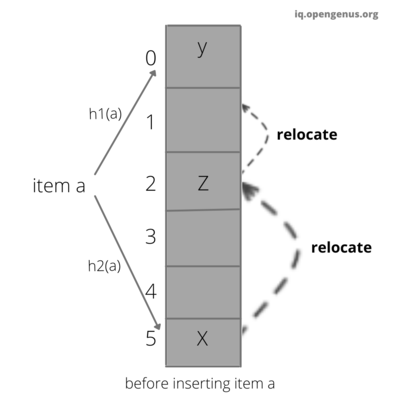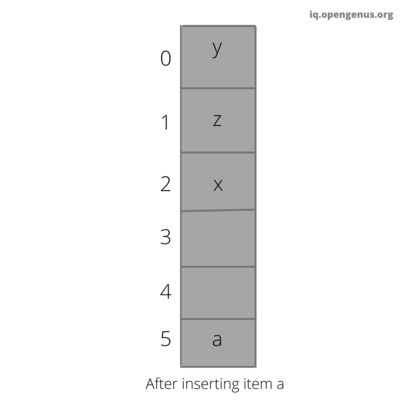# Insertion operation in cuckoo filter

This function is used to insert the value to be stored into the cuckoo filter. It uses cuckoo hashing to insert the value.

### Algorithm implementing insertion in the cuckoo filter.

Step 1: compute the fingerprint of the value to be stored and store it in a variable called fprint.
Step 2: Compute the hash of k(value to be stored), h(k) = kh using the first hash function and store it in a variable called first_bucket.
Step 3: Compute the hash of fprint,h(fprint)=kf
Step 4: Xor first_bucket and hash of fprint and store it in a variable called second_bucket.
Step 5: If first_bucket is empty then insert the fprint in bucket[first_bucket].
Step 6: If first_bucket is not empty then insert the fprint in bucket[second_bucket].
Step 7: If second_bucket is not empty then reallocate the entry present in the second_bucket to empty space and insert the fprint in bucket[second_bucket].

### Pseudocode of insertion function.

``````Insert(x){
fprint=fingerprint(x);
first_bucket=hash(x);
second_bucket=first_bucket⊕hash(fprint);
if bucket[first_bucket] or bucket[second_bucket] has an empty entry then
return Done;

//the two buckets are not empty so we must relocate existing items;
i=randomly pick first_bucket or second_bucket;
for n=0;n<MaxNumKicks;n++ do
randomly select an entry e from bucket[i];
swap fprint and the fingerprint stored in entry e;
i=i⊕hash(fprint);
if bucket[i] has an empty entry then
return Done;

//Hashtable is considered full;
return Failure;
}
``````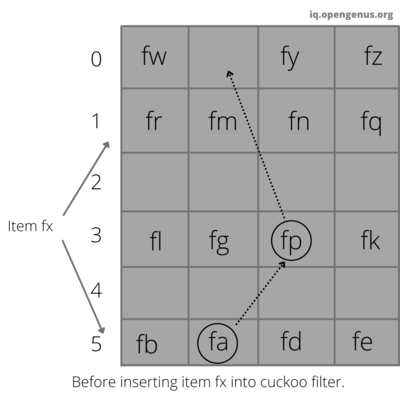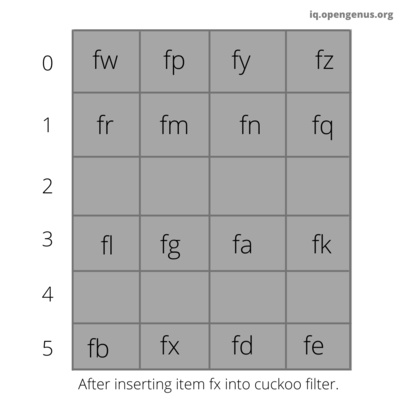# Lookup function in cuckoo filter

Lookup function checks if the given value is present in the cuckoo filter or not. We will compute first_bucket and second_bucket and we will check if the fingerprint of the given value is present in the first_bucket or the second_bucket then it will return true else return false.

### Algorithm implementing lookup in the cuckoo filter.

Step 1: compute the fingerprint of the value to be stored and store it in a variable called fprint.
Step 2: Compute the hash of k(value to be stored), h(k) = kh using the first hash function and store it in a variable called first_bucket.
Step 3: Compute the hash of fprint,h(fprint)=kf
Step 4: Xor first_bucket and hash of fprint and store it in a variable called second_bucket.
Step 5: If bucket[first_bucket] or bucket[second_bucket] contains fprint then return true else return false.

### Pseudocode of lookup function.

``````Lookup(x){
fprint=fingerprint(x);
first_bucket=hash(x);
second_bucket=first_bucket⊕hash(fprint);
if bucket[first_bucket] or bucket[second_bucket] has fprint then
return True;
return False;
``````

# Delete operation in cuckoo filter

Delete function removes a copy of the fingerprint of the given value from the bucket. We will compute first_bucket and second_bucket and we will check if the fingerprint of the given value is present in the first_bucket or the second_bucket then we will remove a copy of fprint from this bucket.

### Algorithm implementing delete in the cuckoo filter.

Step 1: compute the fingerprint of the value to be stored and store it in a variable called fprint.
Step 2: Compute the hash of k(value to be stored), h(k) = kh using the first hash function and store it in a variable called first_bucket.
Step 3: Compute the hash of fprint,h(fprint)=kf
Step 4: Xor first_bucket and hash of fprint and store it in a variable called second_bucket.
Step 5: If bucket[first_bucket] or bucket[second_bucket] contains fprint then remove a copy of fprint from this bucket.

### Pseudocode of Delete function.

``````Delete(x){
fprint=fingerprint(x);
first_bucket=hash(x);
second_bucket=first_bucket⊕hash(fprint);

if bucket[first_bucket] or bucket[second_bucket] has fprint then
remove a copy of fprint from this bucket;
returnTrue;
return False;``````

# Implementing cuckoo filter

Following is the implementation of Cuckoo Filter in GoLang:

• Go

### Go

``````
package main

import (
"bytes"
"encoding/binary"
"errors"
"fmt"
"math"
"crypto/sha1"
"math/rand"
)

type bucket []fingerprint
type fingerprint []byte
var hasher = sha1.New()
const retries = 200 // how many times do we try to move items around?

type Cuckoo struct {
buckets []bucket
m       uint // buckets
bucket_entries       uint // entries per bucket
fingerprint_length       uint // fingerprint length
n       uint // filter capacity
}

func hash(data []byte) []byte {
hasher.Write([]byte(data))
hash := hasher.Sum(nil)
hasher.Reset()
return hash
}

func nextPower(i uint) uint {
i--
i |= i >> 1
i |= i >> 2
i |= i >> 4
i |= i >> 8
i |= i >> 16
i |= i >> 32
i++
return i
}

func fingerprintLength(b uint, e float64) uint {
f := uint(math.Ceil(math.Log(2 * float64(b) / e)))
f /= 8
if f < 1 {
return 1
}
return f// if f is greater than or equal to 1 return f.
}

// n = len(items), fp = false positive rate
func NewCuckoo(n uint, fp float64) *Cuckoo {
bucket_entries := uint(4)
fingerprint_length := fingerprintLength(bucket_entries, fp)
m := nextPower(n / fingerprint_length * 8)
buckets := make([]bucket, m)
for i := uint(0); i < m; i++ {
buckets[i] = make(bucket, bucket_entries)
}
return &Cuckoo{
buckets: buckets,
m:       m,
bucket_entries:  bucket_entries,
fingerprint_length:   fingerprint_length,
n:       n,
}
}

// nextIndex returns the next index for entry, or an error if the bucket is full
func (b bucket) nextIndex() (int, error) {
for i, f := range b {
if f == nil {
return i, nil
}
}
return -1, errors.New("bucket full")
}

// hashes returns h1, h2 and the fingerprint
func (c *Cuckoo) hashes(data string) (uint, uint, fingerprint) {
h := hash([]byte(data))
fprint := h[0:c.fingerprint_length]
first_bucket := uint(binary.BigEndian.Uint32(h))
second_bucket := first_bucket^ uint(binary.BigEndian.Uint32(hash(fprint)))
return first_bucket, second_bucket, fingerprint(fprint)
}

func (b bucket) contains(f fingerprint) (int, bool) {
for i, x := range b {
if bytes.Equal(x, f) {
return i, true
}
}
return -1, false
}

//function that inserts string into the cuckoo filter.
func (c *Cuckoo) insert(input string) {
first_bucket, second_bucket, fprint := c.hashes(input)
// first try bucket one
b1 := c.buckets[first_bucket%c.m]
if i, err := b1.nextIndex(); err == nil {
b1[i] = fprint
return
}
b2 := c.buckets[second_bucket%c.m]
if i, err := b2.nextIndex(); err == nil {
b2[i] = fprint
return
}
// else we need to start relocating items
i := first_bucket
for r := 0; r < retries; r++ {
index := i % c.m
entryIndex := rand.Intn(int(c.bucket_entries))
// swap
fprint, c.buckets[index][entryIndex] = c.buckets[index][entryIndex], fprint
i = i ^ uint(binary.BigEndian.Uint32(hash(fprint)))
b := c.buckets[i%c.m]
if idx, err := b.nextIndex(); err == nil {
b[idx] = fprint
return
}
}
panic("cuckoo filter full")
}

// lookup needle in the cuckoo filter
func (c *Cuckoo) lookup(needle string) bool {
first_bucket, second_bucket, fprint := c.hashes(needle)
_, b1 := c.buckets[first_bucket%c.m].contains(fprint)
_, b2 := c.buckets[second_bucket%c.m].contains(fprint)
return b1 || b2
}

// delete the value from the cuckoo filter
func (c *Cuckoo) delete(needle string) {
first_bucket, second_bucket, fprint := c.hashes(needle)
// try to remove from f1
b1 := c.buckets[first_bucket%c.m]
if ind, ok := b1.contains(fprint); ok {
b1[ind] = nil
return
}

b2 := c.buckets[second_bucket%c.m]
if ind, ok := b2.contains(fprint); ok {
b2[ind] = nil
return
}
}

func main() {
c := NewCuckoo(10, 0.1)
c.insert("hello")
c.insert("world")
ok := c.lookup("world")
fmt.Printf("%v\n", ok)
c.delete("world")
ok = c.lookup("world")
fmt.Printf("%v\n", ok)
} ``````

# Time & Space complexity of Cuckoo Filter

### Time complexity.

• The time complexity of delete is `O(1)` because it does not depend upon the input size.
• The time complexity of lookup is also `O(1)` because it does not depend upon the input size.
• The time complexity of insertion has a reasonably high probability of `O(1)` if the load is well managed.

### Space complexity.

• A cuckoo filter requires ( log2 (1/ϵ) + 2 ) / α bits of space per inserted key

Note:

• `α` is the hash table load factor.The load factor is the average number of key-value pairs per bucket.It is calculated using formula load factor = total number of key-value pairs / number of buckets.
• `ϵ` is the false positive rate.

Example: If the false positive rate is 3% and the load factor is around 95.5% then ϵ is .03 and α is 0.955
substituting these values in the formula
bits of space per inserted key =( log2 (1/0.03) + 2 ) / 0.955
( log2(33.333) + 2 ) / 0.955
( 5.059 + 2 ) / 0.955
7.059 / 0.955
7.391.
So it takes approximately 7 bits per inserted key.

# Advantages of Cuckoo filter over Bloom filter

• It takes less time for lookups.
• The cuckoo filter has fewer false positives than the bloom filter for the same number of items stored.
• It supports the deletion of items.
• It is simple to implement than counting bloom filters.

# Limitation of Cuckoo filter

• It can only delete elements that are known to be inserted before.
• Insertion can fail and rehashing is required like other cuckoo hash tables.

With this article at OpenGenus, you must have the complete idea of Cuckoo Filter. Enjoy. Read more:

#### Raghavendra Achar C

Raghavendra is a student at Ramaiah Institute of Technology and an Intern at OpenGenus.